Our World Statistics Day conversations have been a great reminder of how much statistics can inform our lives. Do you have an example of how statistics has made a difference in your life? Share your story with the Community!
Choose Language Hide Translation Bar
Using JMP to evaluate MCMC diagnostics

It’s no secret that JMP excels in the visual exploration of data. There’s a healthy dose of statistics, too. But when asked about Bayesian methods, JMP is probably not the first software package that comes to mind. JMP 10 does contain Bayesian D-optimal and I-optimal designs in our design of experiments (DOE) features, and Bayesian variance components are available for variability charts. While Bayesian methods may be limited in JMP, it is the perfect tool to evaluate and summarize posterior samples obtained from Monte Carlo Markov Chain (MCMC) estimation.

Let me be clear: JMP does not have methods to fit models in the Bayesian paradigm using (MCMC), but it is valuable for understanding the posterior samples obtained from other packages such as PROC MCMC, WinBUGS or BRugs. All you need is the freely available JMP add-in for MCMC Diagnostics (free SAS profile required).  Below I’ll describe the add-in using posterior samples of 40 adverse event treatment parameters (log-odds ratios) obtained from PROC MCMC using data from a vaccine trial described in Mehrotra & Heyse (2004).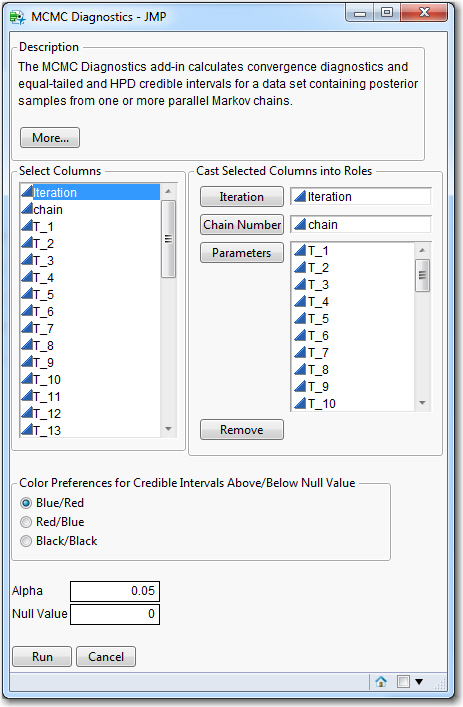Figure 1. JMP MCMC Diagnostics Dialog

The MCMC Diagnostics dialog (Figure 1) displays all of the variables (COLUMNS) of the input data set. The only requirement to run the add-in is that at least one PARAMETER should be specified. In these instances, it is assumed that all samples are from a single Markov chain, and samples will be numbered in trace plots from 1 to the total number of rows in the data set. If ITERATION is provided, trace plots will reflect appropriate sample numbers (say, if burn-in samples were removed). CHAIN specifies a numeric value if multiple Markov chains are generated to assess parameter convergence to the target distribution. COLOR PREFERENCE specifies the color (default Blue/Red) of any credible intervals that exclude the NULL VALUE (default 0). Under the defaults, intervals entirely to the right or left of the null value will be blue or red, respectively. ALPHA (default 0.05) calculates (1-α)˟100% credible intervals for the forest plots.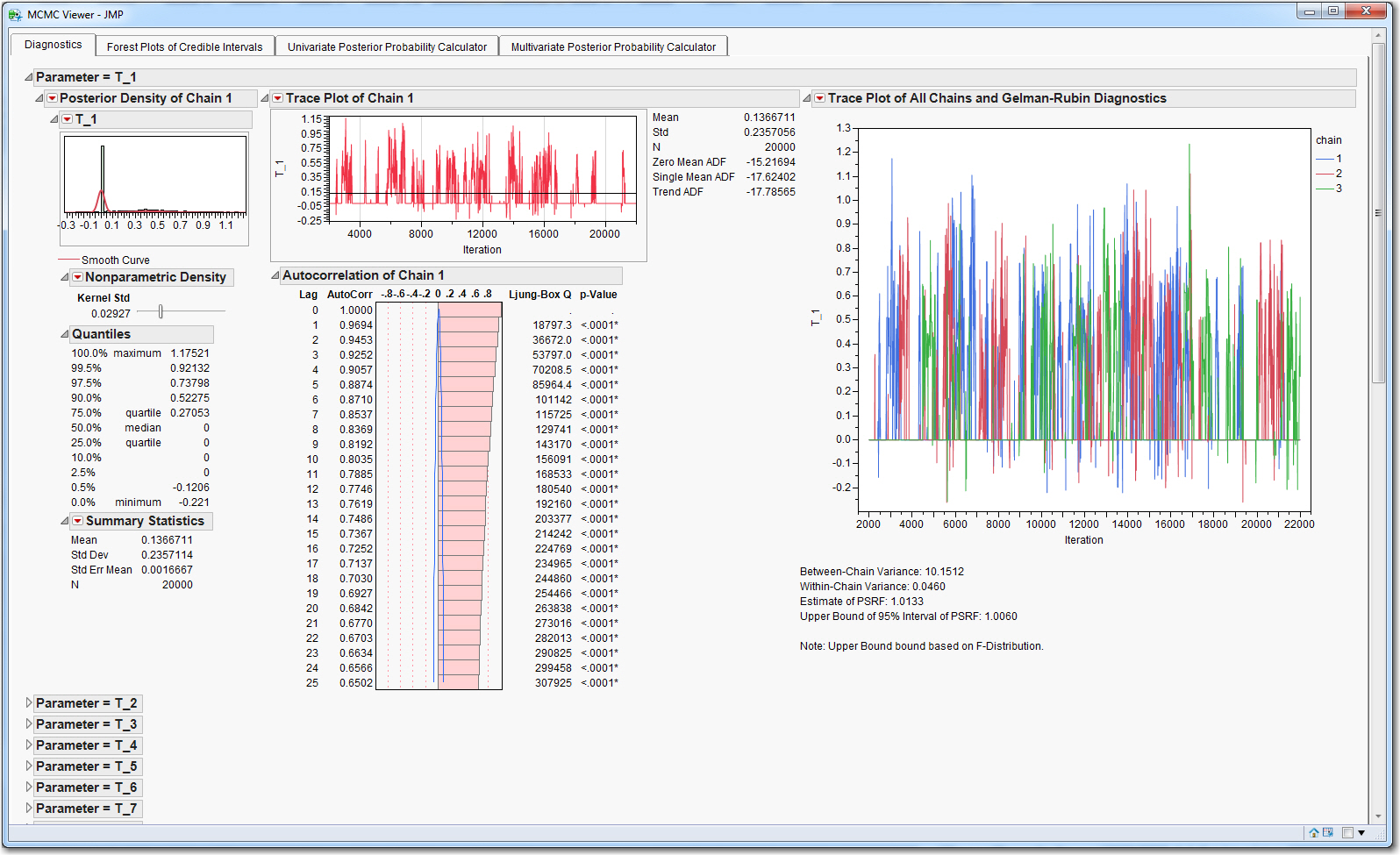Figure 2. MCMC Diagnostics Including Histogram and Density Function of Posterior Samples, Trace Plots, Autocorrelation Assessment and Gelman-Rubin Statistics

The add-in generates the MCMC Viewer Window in Figure 2. The Diagnostics Tab provides histograms, density function curves and summary statistics of the posterior samples from Chain 1 for all parameters. Trace plots summarize the behavior of the Chain 1 samples over the iterations and can be used to assess convergence of the chain to the target distribution. Histograms and summary statistics summarize the autocorrelation of Chain 1 posterior samples up to lag 25. If the analysis includes multiple Markov chains, trace plots summarize all chains simultaneously, and Gelman-Rubin Statistics are provided.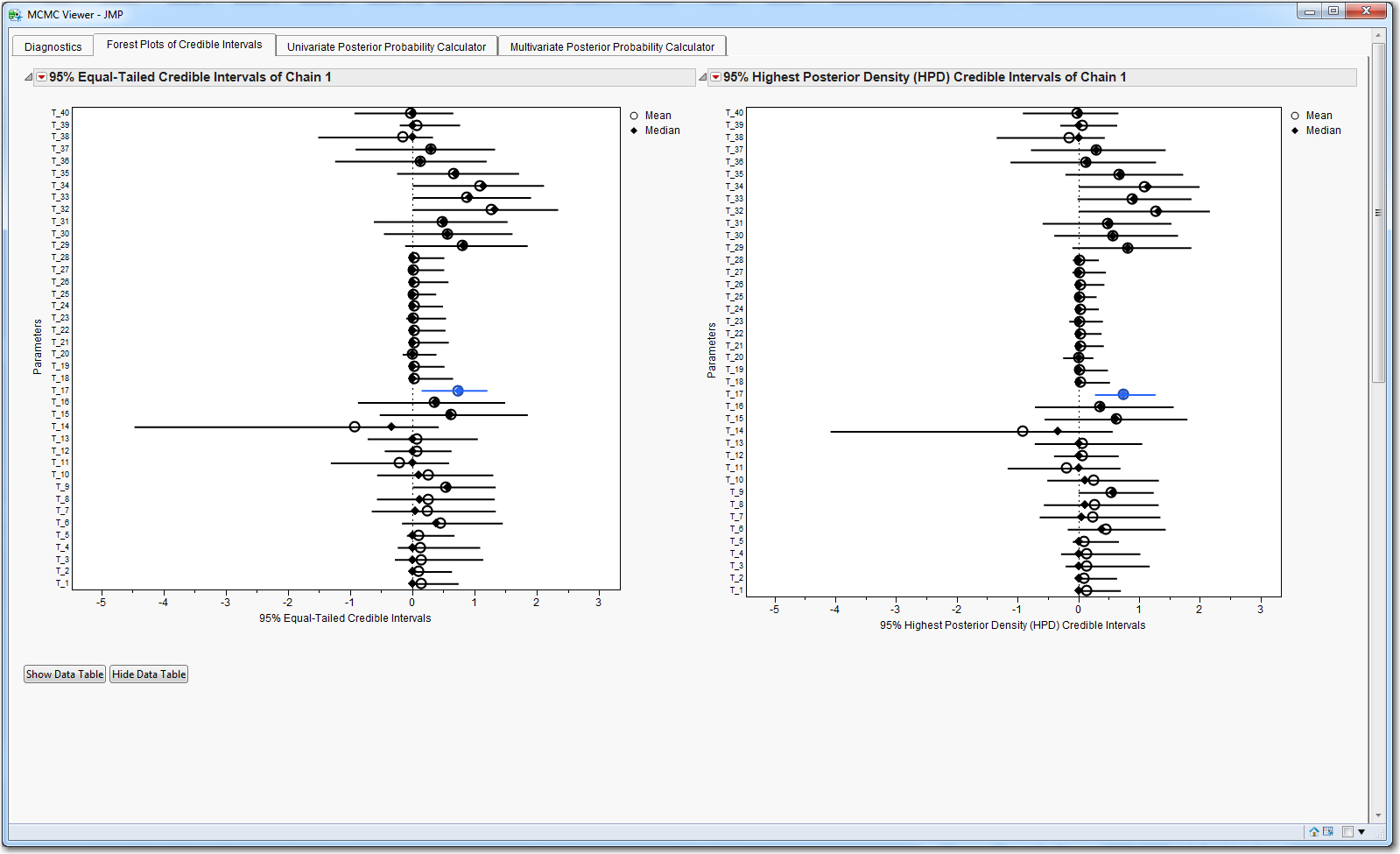Figure 3. 95% Equal-Tailed and HPD Credible Intervals of Posterior Samples

The interactivity of JMP is a key benefit of the add-in. The diagnostic output of all parameters except the first is initially collapsed. This output can be opened or closed by selecting the outline boxes in the Tab. By default, a nonparametric kernel density curve is fit to the posterior samples in the histograms. However, the user can add multiple reference lines from the red triangle menu of the histogram. If needed, a partial autocorrelation or variogram summary figure can be generated from the red triangle menu of any trace plot.

The Forest Plots of Credible Intervals Tab provides two figures of 95% credible intervals for the parameters using samples from Chain 1. Figure 3 summarizes equal-tailed credible intervals of the posterior samples. Here, the lower and upper endpoints for these intervals correspond to the 2.5th and 97.5th percentiles of the samples, respectively. In addition, Figure 3 summarizes the 95% highest posterior density (HPD) credible intervals, which are the narrowest intervals covering 95% of all samples. For both plots, the mean and median sample values are summarized using circles and diamonds, respectively. Only the intervals for T_17, which corresponds to the adverse event of irritability, exclude the assumed null value of 0. If needed, the underlying statistics for these figures are a button-click away.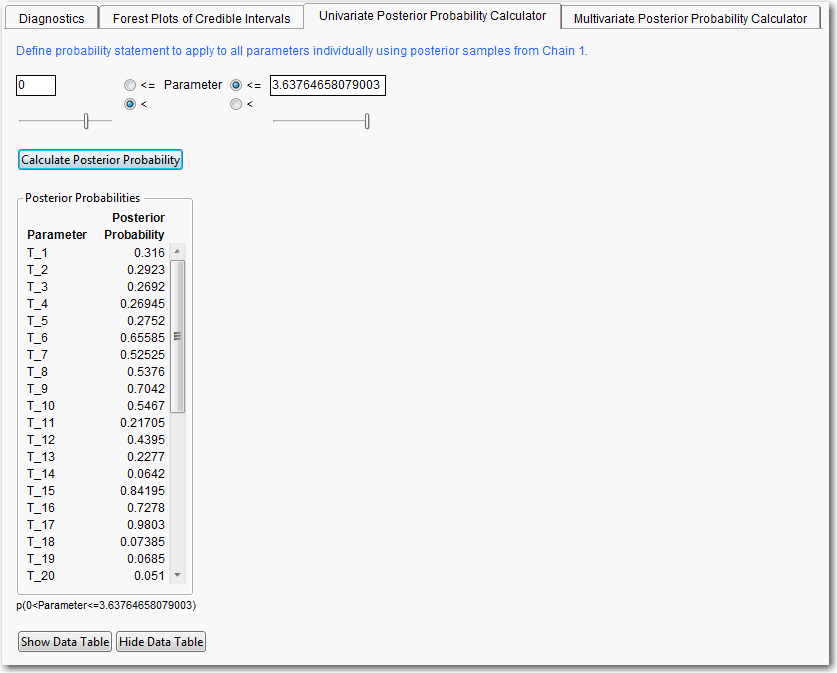Figure 4. Univariate Probability Calculator

The Univariate Posterior Probability Calculator enables the user to define probability statements for the parameters, the results of which are summarized in a table (Figure 4). Ranges can be added manually, or the sliders can be used to select limits which are restricted to the minimum and maximum values of the samples for all parameters from Chain 1.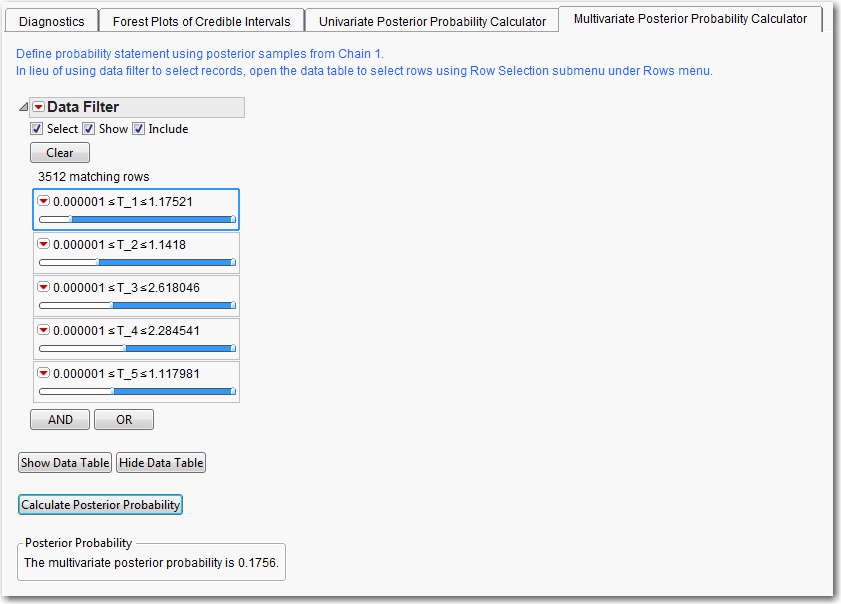Figure 5. Multivariate Probability Calculator

The Multivariate Posterior Probability Calculator lets the user define probability statements that consider two or more parameters simultaneously. Figure 5 illustrates this calculator using only the treatment parameters from the first five adverse events. We calculate the posterior probability that treatment has an undesirable effect (essentially each parameter greater than 0) on astenia/fatigue, fever, infection-fungal, infection-viral and malaise simultaneously as 0.1756. The multivariate calculator makes use of the JMP Data Filter to select data table rows meeting the criteria defined in the filter. Alternatively, the user can open the data table and select rows manually, or apply a function to the columns of interest. Once rows are selected, the user can push the Calculate Posterior Probability button.

Finally, while we analyzed odds ratio parameters on the log-scale, these could have easily been transformed to odds ratios using the Transform function under the Column menu.

Mehrotra, DV & Heyse, JF. (2004). “Use of the False Discovery Rate for Evaluating Clinical Safety Data.” Statistical Methods in Medical Research 13: 227-238.

Article Labels

There are no labels assigned to this post.

Article Tags